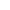# Using the Casio fx-85GT Plus

Using the M+ functionBest Answer pan.gejt , 18 April 2020 - 01:11 PM

1 divided by 3 M+

1 divided by 4 M+

1 divided by 6 M+

Alpha M+ (recall RED M)

the result is 3/4

Go to the full post4 replies to this topic

### #1fx-85GT PLUS

fx-85GT PLUS

Newbie

•• Members
•• 2 posts

Posted 15 April 2020 - 07:18 PM

Hello members and thank you for reading my first post.

I'm not a mathematician by trade so please excuse any mistakes I may write along the way...

Using the above calculator and trying to following some instructions in an auto-electrical book I am asked to use a calculator to solve the fractions;

1/3 + 1/4 + 1/6 = !!!

Now on paper I can work out the denominator 12 and use equivalent fractions to find the answer = 1.3333

The problem is that using the calculator above and the M+ function as asked the calculator does not seem to be able to work out the correct denominator for each fraction and hence cannot reach the correct answer using the instructions as follows;

1 divided by 3 M+

1 divided by 4 M+

1 divided by 6 M+

1 divided  M_RC =

Firstly this casio calculator does not have the function button M_RC but does have RCL if that button performs the same function.

I have the Casio booklet but that booklet also to me does not explain how to use the M+ function so I'm at a loss?

Thank you

### #2Hlib2

Hlib2

•• Members
•• 74 posts
• Gender:Male
• Location:Ukraine
• Interests:industrial electronics,
graphing calculators

• Calculators:
fx-9860gii-2, afx-2.0+
fx-9750gii, fx-9750g+
cfx-9850gb+, graph25
fx-991DE_X, fx-991ES+
ti-voyage200(emulator)
ti-83+_SE, ti-85, hp-50g

Posted 18 April 2020 - 01:02 PM

In the
fx-85GT user`s guide
look for the title "Basic Calculations", where you will find about [M+] function.
• fx-85GT PLUS likes this

### #3pan.gejt

pan.gejt

Casio Freak

•• Members
•• 220 posts
• Gender:Male
• Location:CZ

• Calculators:
.

Posted 18 April 2020 - 01:11 PM   Best Answer

1 divided by 3 M+

1 divided by 4 M+

1 divided by 6 M+

Alpha M+ (recall RED M)

the result is 3/4

• fx-85GT PLUS likes this

### #4fx-85GT PLUS

fx-85GT PLUS

Newbie

•• Members
•• 2 posts

Posted 18 April 2020 - 08:26 PM

Thanks for both replies. After the final 1 divided by M+ I have found that the calculation requires another 1 divided by alpha M = 4/3 = 1.333....

I'd never have got it without your help, thanks

### #5siealex

siealex

Casio Fan

•• Members
•• 41 posts
• Gender:Male

• Calculators:
570w, 570ms, 83es, 570es, 83gt plus, 991es plus, 991de plus, 570spx ii, 9750gii

Posted 13 May 2020 - 09:12 AM

Yes, it's not obvious, but the independent memory "M" in all Casio calculators is the same as the "M" variable. By the way, other manufacturers (especially Citizen) have different ways to process this variable.

#### 0 user(s) are reading this topic

0 members, 0 guests, 0 anonymous users